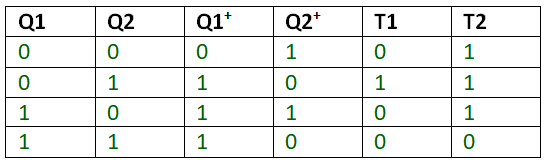# GATE | GATE-CS-2017 (Set 2) | Question 57

The next state table of a 2 bit saturating up-counter is given below.The counter is built as synchronous sequential circuit using T flip-flops. The value for T1 and T0 are
(A) T1 = Q0Q1
T0 = Q’0Q’1
(B) T1 = Q’1Q0
T0 = Q’1 + Q’0
(C) T1 = Q1 + Q0
T0 = Q’1 + Q’0
(D) T1 = Q’1Q0
T0 = Q1 + Q0

Explanation:

T1 = Q’1Q0 = 0100
T0 = Q’1 + Q’0 = 1110

Therefore Option B

Another SolutionT1 and T2 are filled by using the property that output of T FF will change when T=1 and will not change when T=0
Thus

```T1(Q1,Q2) = Q1’ Q2
T2(Q1,Q2) = Q1’Q2’ + Q1’Q2 + Q1Q2’
= Q1’ + Q1Q2’
= Q1’ + Q2’
```

This solution is contributed by Abhishek Kumar.

Quiz of this Question

My Personal Notes arrow_drop_up
Article Tags :

Be the First to upvote.

Please write to us at contribute@geeksforgeeks.org to report any issue with the above content.